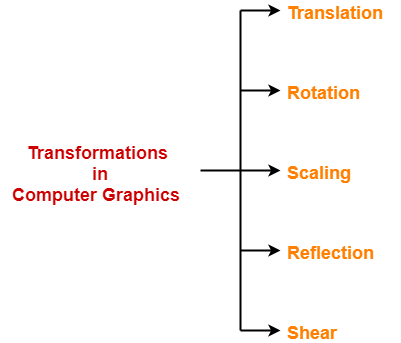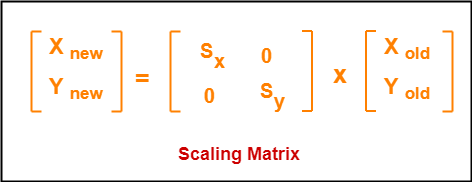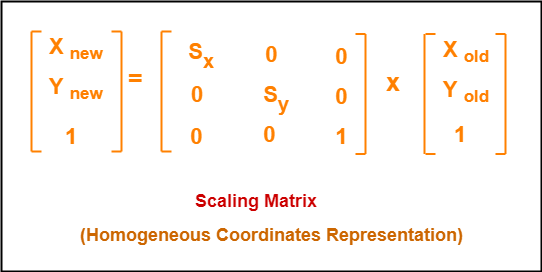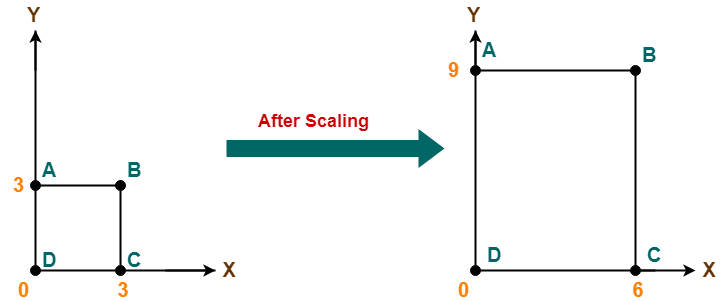## 2D Transformations in Computer Graphics-

We have discussed-

• Transformation is a process of modifying and re-positioning the existing graphics.
• 2D Transformations take place in a two dimensional plane.

In computer graphics, various transformation techniques are-## 2D Scaling in Computer Graphics-

 In computer graphics, scaling is a process of modifying or altering the size of objects.

• Scaling may be used to increase or reduce the size of object.
• Scaling subjects the coordinate points of the original object to change.
• Scaling factor determines whether the object size is to be increased or reduced.
• If scaling factor > 1, then the object size is increased.
• If scaling factor < 1, then the object size is reduced.

Consider a point object O has to be scaled in a 2D plane.

Let-

• Initial coordinates of the object O = (Xold, Yold)
• Scaling factor for X-axis = Sx
• Scaling factor for Y-axis = Sy
• New coordinates of the object O after scaling = (Xnew, Ynew)

This scaling is achieved by using the following scaling equations-

• Xnew = Xold x Sx
• Ynew = Yold x Sy

In Matrix form, the above scaling equations may be represented as-For homogeneous coordinates, the above scaling matrix may be represented as a 3 x 3 matrix as-## Problem-01:

Given a square object with coordinate points A(0, 3), B(3, 3), C(3, 0), D(0, 0). Apply the scaling parameter 2 towards X axis and 3 towards Y axis and obtain the new coordinates of the object.

## Solution-

Given-

• Old corner coordinates of the square = A (0, 3), B(3, 3), C(3, 0), D(0, 0)
• Scaling factor along X axis = 2
• Scaling factor along Y axis = 3

### For Coordinates A(0, 3)

Let the new coordinates of corner A after scaling = (Xnew, Ynew).

Applying the scaling equations, we have-

• Xnew = Xold x Sx = 0 x 2 = 0
• Ynew = Yold x Sy = 3 x 3 = 9

Thus, New coordinates of corner A after scaling = (0, 9).

### For Coordinates B(3, 3)

Let the new coordinates of corner B after scaling = (Xnew, Ynew).

Applying the scaling equations, we have-

• Xnew = Xold x Sx = 3 x 2 = 6
• Ynew = Yold x Sy = 3 x 3 = 9

Thus, New coordinates of corner B after scaling = (6, 9).

### For Coordinates C(3, 0)

Let the new coordinates of corner C after scaling = (Xnew, Ynew).

Applying the scaling equations, we have-

• Xnew = Xold x Sx = 3 x 2 = 6
• Ynew = Yold x Sy = 0 x 3 = 0

Thus, New coordinates of corner C after scaling = (6, 0).

### For Coordinates D(0, 0)

Let the new coordinates of corner D after scaling = (Xnew, Ynew).

Applying the scaling equations, we have-

• Xnew = Xold x Sx = 0 x 2 = 0
• Ynew = Yold x Sy = 0 x 3 = 0

Thus, New coordinates of corner D after scaling = (0, 0).

Thus, New coordinates of the square after scaling = A (0, 9), B(6, 9), C(6, 0), D(0, 0).To gain better understanding about 2D Scaling in Computer Graphics,

Watch this Video Lecture

Next Article- 2D Reflection in Computer Graphics

Get more notes and other study material of Computer Graphics.

Watch video lectures by visiting our YouTube channel LearnVidFun.